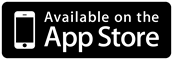## Maths Investigation: Archery scores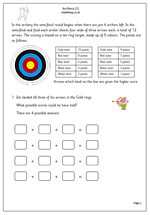Archery is usually organised as a knockout competition with the last two archers left going for the First and Second place medals.

The target consists of ten rings, made up of 5 colours. Points are scored depending on which ring the arrow lands. Arrows which land on the line are given the higher score. 10 points are rewarded for a Gold Inner, 9 for a Gold Outer, down to 2 points for a White Inner and one point for a White Outer.

This worksheet asks what possible scores can be achieved by landing all three arrows in the Gold Rings.

The second page asks what scores are possible if one arrow lands in Gold, one in Red and one in Blue. There are 8 combinations, with just four possible totals.

This could easily be extended to find other possible combinations.

This page is best suityed to Year3/4 children and can be found in our Year 4 Using and Applying Maths category.

Archery investigation (1)

## Year 3 Mental Arithmetic: Sets 67 and 68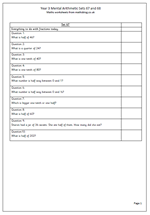We will be shortly be drawing to a close the weekly year 3 mental arithmetic. Meanwhile here is a set of questions concentrating on fractions. Always a tricky subject, often disliked by children and adults, they can, in fact, be quite good fun.

By the end of year 3 children should know that dividing by 2 will give half of a number; dividing by 4 will give a quarter of a number and dividing by 10 will give a tenth of a number.

One of the puzzling things about fractions can be that as a number gets bigger, the fraction gets smaller. So 1/10 of a number is a smaller amount than 1/4 or 1/6 of that number.

Something else which many children find tricky is finding the number half way between two other numbers, such as finding the number half way between 8 and a half and nine. Plenty of practice counting in halves and quarters will help with this.

Year 3 mental arithmetic: sets 67 and 68

## Written Addition worksheets: 2-digits to 3-digits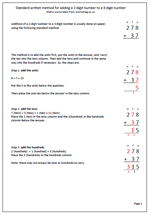Addition of a 2-digit number to a 3-digit number is usually done on paper, using the following standard method:

The method is to add the units first, put the units in the answer, and ‘carry’ the ten into the tens column. Then add the tens and continue in the same way into the hundreds if necessary. So, looking at the addition 278 +37

8 + 7 = 15

Put the 5 in the units below the question.

Then place the one ten below the answer in the tens column.

7 (tens) + 3 (tens) + 1 (ten) = 11 (tens)

Place the 1 (ten) in the tens column and the 1(hundred) in the hundreds

2 (hundreds)  + 1 (hundred) = 3 (hundreds)

Place the 3 (hundreds) in the hundreds column.

Note: there may not always be tens or hundreds to carry.

This type of question is good for children who are not confident with the standard written method of addition.

Standard addition of 2-digits to 3-digits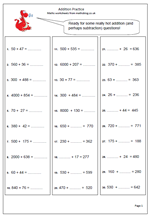Here we have an excellent page to check how well children can deal with different types of addition questions, some of which are most easily answered by subtracting! By year 4 we are looking for rapid responses using a variety of different skills depending on the type of question. Children need to look at the question and make a very quick decision about how best to tackle it. Sometimes they may want to make jottings to assist with remembering what they have already done.

Let’s look at some of the different types of question and some possible approaches.

a. 400 + 345. An instant recognition that just the hundreds need to be added and the tens and units will remain the same. Should be very quick.

b. 650 + 18. This is probably best done by ‘adding on’ add 10 to 650 makes 660 plus the 8 making 668.

c. 430 + ??? = 580. Either done as a subtraction taking 430 from 580, or by adding on. If doing the subtraction it is quite usual to start with the hundreds 500 take 400 is 100; then the tens 80 take 30 is 50; answer 150. (The reverse of doing this on paper.) If adding on children may well start with adding either the hundreds or the tens as both are equally good.

d. ??? + 50 = 460. Again this can be done by adding on from 50. Add 10 to make 60. Add 400 to make 460. Answer 110. It can also be done just as quickly by subtracting 50 from 460.

## Year 3 Mental Arithmetic: Sets 65 and 66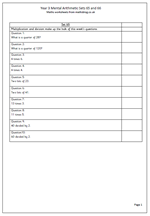This week the year 3 mental arithmetic questions are all about multiplication and division, including finding quarters. When asked to find a quarter of a number often one of the best approaches is to halve the number and halve again.This works best with even numbers

Some of the multiplication questions look at knowledge of tables, such as 4 times 4, but some of the later questions require this knowledge to be put into use to work out mentally some trickier questions, such as 13 times 3.

There are several ways that 13 times 3 can be approached. One way is to use known facts such as 10 x 3 is 30 and 3 x 3 is 9. Another way is to double 13, making 26 and then adding another 13 to get to 39. Once again it is worth saying that asking children how they reach an answer reveals an awful lot about their knowledge of maths. Unfortunately teachers in a class of 30 children do not have the time to do this with each individual child so it is important to do it at home!

Year 3 mental arithmetic: sets 65 and 66

## Mental Division Strategies: year 4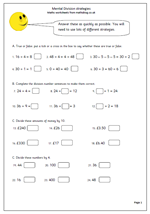Having a browse round the site there seemed to be some areas which are a little short of pages; one of these being the year 4 division pages. So, here is a Year 4 division worksheet which looks at some of the strategies children need to be confident with in order to carry out quick, efficient division calculations.

The worksheet is divided into 4 sections, each with a different purpose. The first set of questions looks at basic concepts, such as dividing by 1 and the fact that, unlike addition the calculation has to be carried out in the order stated e.g. 34 divided by 2 is not the same as 2 divided by 34.

The second set of questions takes a quick look at division facts. Many teachers are now of the opinion that division facts should be learned, just like multiplication facts (tables.) This can be argued with in that if a child knows all the times tables they can quickly use them to work out division problems.

The third set looks at dividing money by 10. Of course this is easily done by moving each digit one place to the right. As it is money there should be 2 digits after the decimal point. e.g. £32 divided by 10 should be £3.20. Watch out for those children who get confused because they have been told to ‘add a nought’ when multiplying by 10. Never a good idea!

The last set is all about dividing by 4. Often this can be done by halving and then halving again.

Mental division strategies

## Year 5 Maths Challenge: the very best 2-digit answers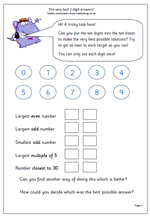This is quite an intriguing worksheet as it is difficult to decide if there is a best possible answer. In fact, part of the challenge could be to decide what the criteria are for deciding what counts as the best possible answer.

The idea is to use each of the digits 0 to 9 just once each to fulfil the statements below. So, for example 98 could be used for the largest even number and 67 used as the largest odd number. But is this the best option? Would 86 and 97 be better?

One which does have to be considered early on is the multiple of five as this ensures either the digit 5 or the digit 0 are used.

A good worksheet to generate discussion and with no answer it is entirely up to you!

This page can be found in the Year 5 Using and Applying Maths category.

## Year 3 mental arithmetic: sets 63 and 64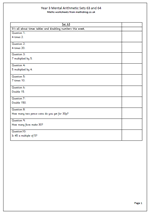We continue into the second part of the summer term with our next set of year 3 mental arithmetic questions. This week’s questions are particularly suitable for reading out loud, rather than printing and looking at, as they are all short. They concentrate on times tables, doubling and division.

The questions on times tables concentrate on the two, times, five times and ten times tables which children should know ‘off by heart’ by this stage. Question 2 is trickier as it needs to make use of the 2 times table to multiply by 20. The doubling questions look at doubling multiples of 5.

Question ten uses the term multiple which children should have come across this year, probably in terms of the answers to times tables i.e. the product of two whole numbers.

All the mental arithmetic sets of questions can be found in the Year 2 Calculating category.

Year 3 mental arithmetic_(sets 63 and 64)

## Daft Draft National Curriculum changes in maths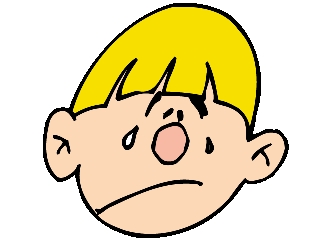Well, I was going to let this pass by with no comment, but on reading it I  am becoming more and more certain that it has been written by idiots who last visited a primary school in 1961.

Firstly, it expects children to ‘use a compass to draw circles and arcs with a given radius’ in year 3. Hard enough with a pair of compasses but impossible with a compass which I use to find directions!

Secondly, children will have to learn tables up to 12, although we did away with 12 pence to a shilling many, many years ago. Whilst I have no problem with children knowing this I fail to understand why they need it.

Thirdly, ‘childen’ are no longer mentioned; they have become impersonal ‘pupils’ throughout.

Oh, and why has addition and subtraction of money suddenly become part of measurement and not part of addition and subtraction?

Rant over.

## Maths Champ for iPhone & iPad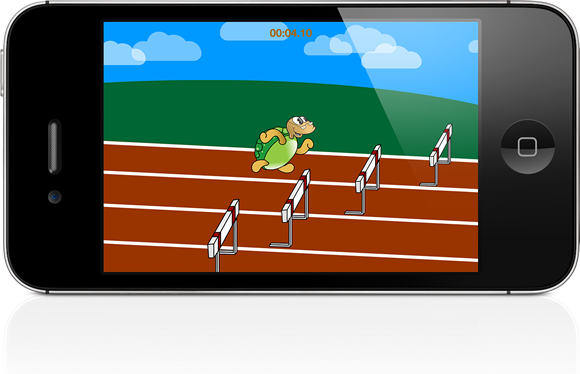# Be a Maths Champ!

I have just played a great new iPhone and iPad game Maths Champ.

There are a couple of mini games included, Hurdles and Javelin. Each time you play, you answer a set of maths questions which boosts your speed or distance when playing the game and gives you a better chance of achieving a Gold medal.

It’s not easy to find apps with a combination of maths  and fun games skills, but this is certainly challenging and fun. It gives excellent practice at mental maths and could really improve your mental maths skills – I have really struggled to achieve Gold on the hard level!

Maths Champ is a universal app with iPhone and iPad support. It looks great and support the normal and retina display of both the iPhone and iPad.

Currently it is on a launch offer, so get it whilst it’s cheap!